# Number of Isosceles triangles in a binary tree

Pre-Requisites: Depth First Search | Parent Array Representation

Given a parent array representation of a binary tree, we need to find the number of Isosceles triangles in the binary tree.

Consider a parent array representing a binary tree:
Parent Array: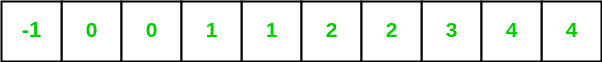Given below is the tree representation of the given parent array.
Binary Tree: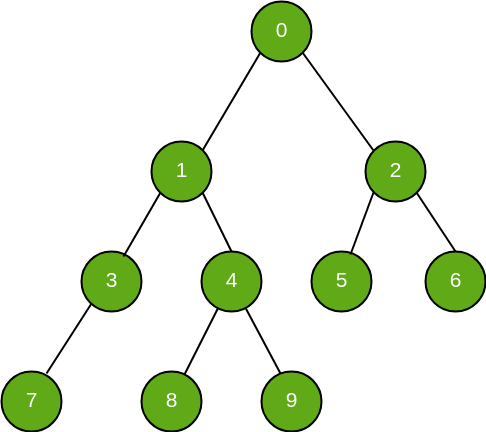There are three types of isosceles triangles which can be found inside a binary tree. These three different types of isosceles triangles can be handled as three different cases.

Case 1: Apex(Vertex against the base sharing equal sides) has two successors(both direct/indirect).
This case can be represented as: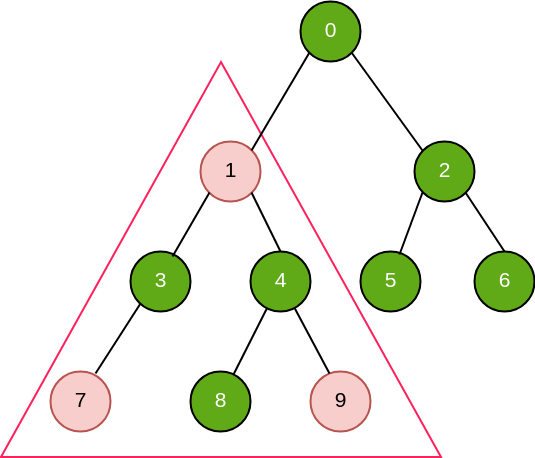In the given tree, there are 6 such isosceles triangles i.e; (0, 1, 2), (0, 3, 6), (1, 3, 4), (1, 7, 9), (4, 8, 9), (2, 5, 6)

`Pseudo Code: `count += min(left_down[i], right_down[i]);``

Case 2: Apex has a left successor(direct/indirect) and apex itself is a right successor(direct/indirect) of its parent.
This case can be represented as: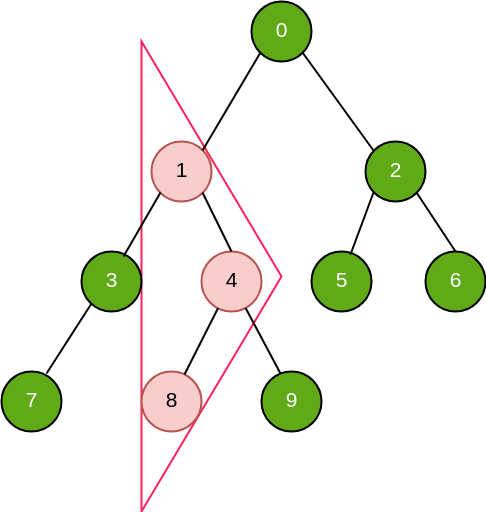In the given tree, there are 2 such isosceles triangles i.e; (1, 8, 4), (0, 5, 2)

`Pseudo Code: `count += min(left_down[i], left_up[i]);``

Case 3: Apex has a right successor(direct/indirect) and apex itself is a left successor(direct/indirect) of its parent.
This case can be represented as: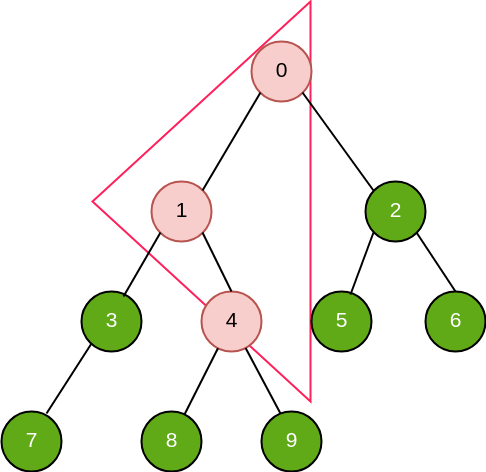In the given tree, there is 1 such isosceles triangle i.e; (0, 1, 4)

`Pseudo Code: `count += min(right_down[i], right_up[i]);``

Pseudo Code legend:
left_down[i] -> maximum distance of ith node from its farthest left successor
right_down[i] -> maximum distance of ith node from its farthest right successor
left_up[i] -> maximum distance of ith node from its farthest left predecessor
right_up[i] -> maximum distance of ith node from its farthest right predecessor

Below is the implementation to calculate the number of isosceles triangles present in a given binary tree:

## C++

 `/* C++ program for calculating number of  ` `isosceles triangles present in a binary tree */` `#include ` `using` `namespace` `std; ` ` `  `#define MAX_SZ int(1e5) ` ` `  `/* Data Structure used to store  ` `   ``Binary Tree in form of Graph */` `vector<``int``>* graph; ` ` `  `// Data varirables ` `int` `right_down[MAX_SZ]; ` `int` `left_down[MAX_SZ]; ` `int` `right_up[MAX_SZ]; ` `int` `left_up[MAX_SZ]; ` ` `  `/* Utility function used to  ` `   ``start a DFS traversal over a node */` `void` `DFS(``int` `u, ``int``* parent) ` `{ ` ` `  `    ``if` `(graph[u].size() != 0) ` `        ``sort(graph[u].begin(), graph[u].end()); ` ` `  `    ``if` `(parent[u] != -1) { ` `        ``if` `(graph[parent[u]].size() > 1) { ` `            ``/* check if current node is  ` `                                ``left child of its parent */` `            ``if` `(u == graph[parent[u]]) { ` `                ``right_up[u] += right_up[parent[u]] + 1; ` `            ``} ` `            ``// current node is right child of its parent ` `            ``else` `{ ` `                ``left_up[u] += left_up[parent[u]] + 1; ` `            ``} ` `        ``} ` `        ``/* check if current node is left and  ` `                            ``only child of its parent */` `        ``else` `{ ` `            ``right_up[u] += right_up[parent[u]] + 1; ` `        ``} ` `    ``} ` `    ``for` `(``int` `i = 0; i < graph[u].size(); ++i) { ` ` `  `        ``int` `v = graph[u][i]; ` ` `  `        ``// iterating over subtree ` `        ``DFS(v, parent); ` ` `  `        ``// left child of current node ` `        ``if` `(i == 0) { ` `            ``left_down[u] += left_down[v] + 1; ` `        ``} ` `        ``// right child of current node ` `        ``else` `{ ` `            ``right_down[u] += right_down[v] + 1; ` `        ``} ` `    ``} ` `} ` ` `  `/* utility function used to generate  ` `                ``graph from parent array */` `int` `generateGraph(``int``* parent, ``int` `n) ` `{ ` ` `  `    ``int` `root; ` ` `  `    ``graph = ``new` `vector<``int``>[n]; ` ` `  `    ``// Generating graph from parent array ` `    ``for` `(``int` `i = 0; i < n; ++i) { ` ` `  `        ``// check for non-root node ` `        ``if` `(parent[i] != -1) { ` `            ``/* creating an edge from node with number ` `             ``parent[i] to node with number i */` `            ``graph[parent[i]].push_back(i); ` `        ``} ` `        ``// initializing root ` `        ``else` `{ ` `            ``root = i; ` `        ``} ` ` `  `        ``// Initializing necessary data variables ` `        ``left_up[i] = 0; ` `        ``right_up[i] = 0; ` `        ``left_down[i] = 0; ` `        ``right_down[i] = 0; ` `    ``} ` `    ``// root of the binary tree ` `    ``return` `root; ` `} ` ` `  `// Driver Function ` `int` `main() ` `{ ` ` `  `    ``int` `n = 10; ` ` `  `    ``/* Parent array used for storing  ` `       ``parent of each node */` `    ``int` `parent[] = { -1, 0, 0, 1, 1, 2, 2, 3, 4, 4 }; ` ` `  `    ``/* generateGraph() function generates a graph a  ` `       ``returns root of the graph which can be used for ` `       ``starting DFS traversal */` `    ``int` `root = generateGraph(parent, n); ` ` `  `    ``// triggering dfs for traversal over graph ` `    ``DFS(root, parent); ` ` `  `    ``int` `count = 0; ` ` `  `    ``// Calculation of number of isosceles triangles ` `    ``for` `(``int` `i = 0; i < n; ++i) { ` `        ``count += min(right_down[i], right_up[i]); ` `        ``count += min(left_down[i], left_up[i]); ` `        ``count += min(left_down[i], right_down[i]); ` `    ``} ` ` `  `    ``cout << ``"Number of isosceles triangles "` `         ``<< ``"in the given binary tree are "` `<< count; ` ` `  `    ``return` `0; ` `} `

## Java

 `/* JAVA program for calculating number of  ` `isosceles triangles present in a binary tree */` ` `  `import` `java.io.*; ` `import` `java.util.*; ` ` `  `@SuppressWarnings``(``"unchecked"``) ` `class` `Isosceles_triangles { ` ` `  `    ``static` `int` `MAX_SZ = (``int``)1e5; ` ` `  `    ``/* Data Structure used to store  ` `       ``Binary Tree in form of Graph */` `    ``static` `ArrayList[] graph; ` ` `  `    ``// Data varirables ` `    ``static` `int``[] right_down = ``new` `int``[MAX_SZ]; ` `    ``static` `int``[] left_down = ``new` `int``[MAX_SZ]; ` `    ``static` `int``[] right_up = ``new` `int``[MAX_SZ]; ` `    ``static` `int``[] left_up = ``new` `int``[MAX_SZ]; ` ` `  `    ``/* Utility function used to  ` `       ``start a DFS traversal over a node */` `    ``public` `static` `void` `DFS(``int` `u, ``int``[] parent) ` `    ``{ ` ` `  `        ``if` `(graph[u] != ``null``) ` `            ``Collections.sort(graph[u]); ` ` `  `        ``if` `(parent[u] != -``1``) { ` `            ``if` `(graph[parent[u]].size() > ``1``) { ` `                ``/* check if current node is  ` `                                ``left child of its parent */` `                ``if` `(u == graph[parent[u]].get(``0``)) { ` `                    ``right_up[u] += right_up[parent[u]] + ``1``; ` `                ``} ` `                ``// current node is right child of its parent ` `                ``else` `{ ` `                    ``left_up[u] += left_up[parent[u]] + ``1``; ` `                ``} ` `            ``} ` `            ``/* check if current node is left and  ` `                                ``only child of its parent */` `            ``else` `{ ` `                ``right_up[u] += right_up[parent[u]] + ``1``; ` `            ``} ` `        ``} ` ` `  `        ``if` `(graph[u] == ``null``) ` `            ``return``; ` ` `  `        ``for` `(``int` `i = ``0``; i < graph[u].size(); ++i) { ` ` `  `            ``int` `v = graph[u].get(i); ` ` `  `            ``// iterating over subtree ` `            ``DFS(v, parent); ` ` `  `            ``// left child of current node ` `            ``if` `(i == ``0``) { ` `                ``left_down[u] += left_down[v] + ``1``; ` `            ``} ` `            ``// right child of current node ` `            ``else` `{ ` `                ``right_down[u] += right_down[v] + ``1``; ` `            ``} ` `        ``} ` `    ``} ` ` `  `    ``static` `int` `min(Integer a, Integer b) ` `    ``{ ` `        ``return` `(a < b) ? a : b; ` `    ``} ` ` `  `    ``/* utility function used to generate  ` `                    ``graph from parent array */` `    ``public` `static` `int` `generateGraph(``int``[] parent, ``int` `n) ` `    ``{ ` ` `  `        ``int` `root = -``1``; ` ` `  `        ``graph = (ArrayList[]) ``new` `ArrayList[n]; ` ` `  `        ``// Generating graph from parent array ` `        ``for` `(``int` `i = ``0``; i < n; ++i) { ` ` `  `            ``// check for non-root node ` `            ``if` `(parent[i] != -``1``) { ` `                ``/* creating an edge from node with number ` `                 ``parent[i] to node with number i */` `                ``if` `(graph[parent[i]] == ``null``) { ` `                    ``graph[parent[i]] = ``new` `ArrayList(); ` `                ``} ` `                ``graph[parent[i]].add(i); ` `                ``// System.out.println(graph); ` `            ``} ` `            ``// initializing root ` `            ``else` `{ ` `                ``root = i; ` `            ``} ` ` `  `            ``// Initializing necessary data variables ` `            ``left_up[i] = ``0``; ` `            ``right_up[i] = ``0``; ` `            ``left_down[i] = ``0``; ` `            ``right_down[i] = ``0``; ` `        ``} ` `        ``// root of the binary tree ` `        ``return` `root; ` `    ``} ` ` `  `    ``// Driver Function ` `    ``public` `static` `void` `main(String[] args) ` `    ``{ ` ` `  `        ``int` `n = ``10``; ` ` `  `        ``/* Parent array used for storing  ` `           ``parent of each node */` `        ``int``[] parent = ``new` `int``[] { -``1``, ``0``, ``0``, ``1``, ``1``, ``2``, ``2``, ``3``, ``4``, ``4` `}; ` ` `  `        ``/* generateGraph() function generates a graph a  ` `           ``returns root of the graph which can be used for ` `           ``starting DFS traversal */` `        ``int` `root = generateGraph(parent, n); ` ` `  `        ``// System.exit(0); ` ` `  `        ``// triggering dfs for traversal over graph ` `        ``DFS(root, parent); ` ` `  `        ``int` `count = ``0``; ` ` `  `        ``// Calculation of number of isosceles triangles ` `        ``for` `(``int` `i = ``0``; i < n; ++i) { ` `            ``count += min(right_down[i], right_up[i]); ` `            ``count += min(left_down[i], left_up[i]); ` `            ``count += min(left_down[i], right_down[i]); ` `        ``} ` `        ``System.out.println(``"Number of isosceles triangles "` `                           ``+ ``"in the given binary tree are "` `                           ``+ Integer.toString(count)); ` ` `  `        ``System.exit(``0``); ` `    ``} ` `} `

## Python3

 `''' Python3 program for calculating number of  ` `isosceles triangles present in a binary tree '''` ` `  `MAX_SZ ``=` `int``(``1e5``) ` ` `  `''' Data Structure used to store  ` `  ``Binary Tree in form of Graph '''` `graph ``=` `{} ` ` `  `# Data varirables  ` `right_down ``=` `MAX_SZ``*``[``0``] ` `left_down ``=` `MAX_SZ``*``[``0``] ` `right_up ``=` `MAX_SZ``*``[``0``] ` `left_up ``=` `MAX_SZ``*``[``0``] ` ` `  `''' Utility function used to  ` `    ``start a DFS traversal over a node '''` `def` `DFS(u, parent): ` ` `  `    ``if` `u ``in` `graph: ` `        ``graph[u].sort() ` ` `  `    ``if` `parent[u] !``=` `-``1``: ` `        ``if` `u ``in` `graph ``and` `len``(graph[parent[u]]) > ``1``: ` `            ``''' check if current node is  ` `                            ``left child of its parent '''` `            ``if` `u ``=``=` `graph[parent[u]][``0``] : ` `                ``right_up[u] ``+``=` `right_up[parent[u]] ``+` `1` `             `  `            ``# current node is right child of its parent ` `            ``else``: ` `                ``left_up[u] ``+``=` `left_up[parent[u]] ``+` `1` ` `  `        ``else` `: ` `            ``''' check if current node is left and  ` `                            ``only child of its parent '''` `            ``right_up[u] ``+``=` `right_up[parent[u]] ``+` `1` `         `  `    ``if` `u ``in` `graph: ` `        ``for` `i ``in` `range``(``0``, ``len``(graph[u])): ` ` `  `            ``v ``=` `graph[u][i] ` ` `  `            ``# iterating over subtree ` `            ``DFS(v, parent) ` ` `  `            ``# left child of current node ` `            ``if` `i ``=``=` `0``: ` `                ``left_down[u] ``+``=` `left_down[v] ``+` `1``; ` `             `  `            ``# right child of current node ` `            ``else``: ` `                ``right_down[u] ``+``=` `right_down[v] ``+` `1``; ` ` `  ` `  `''' utility function used to generate  ` `                ``graph from parent array '''` `def` `generateGraph(parent, n): ` `     `  `    ``root ``=` `-``1` ` `  `    ``# Generating graph from parent array ` `    ``for` `i ``in` `range``(``0``, n): ` `         `  `        ``# check for non-root node ` `        ``if` `parent[i] !``=` `-``1``: ` `            ``''' creating an edge from node with number ` `             ``parent[i] to node with number i '''` `            ``if` `parent[i] ``not` `in` `graph: ` `                ``graph[parent[i]] ``=` `[i] ` `            ``else` `: ` `                ``graph[parent[i]].append(i) ` `         `  `        ``# initializing root ` `        ``else` `: ` `            ``root ``=` `i ` `     `  `    ``# root of the binary tree ` `    ``return` `root; ` ` `  `# Driver Function ` `if` `__name__ ``=``=` `'__main__'``: ` ` `  `    ``n ``=` `10` ` `  `    ``''' Parent array used for storing  ` `       ``parent of each node '''` `    ``parent ``=` `[``-``1``, ``0``, ``0``, ``1``, ``1``, ``2``, ``2``, ``3``, ``4``, ``4``] ` ` `  `    ``''' generateGraph() function generates a graph a  ` `    ``returns root of the graph which can be used for ` `     ``starting DFS traversal '''` `    ``root ``=` `generateGraph(parent, n) ` `         `  `    ``# triggering dfs for traversal over graph ` `    ``DFS(root, parent) ` ` `  `    ``count ``=` `0` ` `  `    ``# Calculation of number of isosceles triangles ` `    ``for` `i ``in` `range``(``0``, n): ` `        ``count ``+``=` `min``(right_down[i], right_up[i]) ` `        ``count ``+``=` `min``(left_down[i], left_up[i]) ` `        ``count ``+``=` `min``(left_down[i], right_down[i]) ` `     `  `    ``print``(``"Number of isosceles triangles "`  `            ``+` `"in the given binary tree are "`  `            ``+` `str``(count)) `

## C#

 `/* C# program for calculating number of  ` `isosceles triangles present in a binary tree */` `using` `System; ` `using` `System.Collections.Generic; ` `using` `System.Linq; ` ` `  `class` `Isosceles_triangles ` `{ ` ` `  `    ``static` `int` `MAX_SZ = (``int``)1e5; ` ` `  `    ``/* Data Structure used to store  ` `    ``Binary Tree in form of Graph */` `    ``static` `List<``int``>[] graph; ` ` `  `    ``// Data varirables ` `    ``static` `int``[] right_down = ``new` `int``[MAX_SZ]; ` `    ``static` `int``[] left_down = ``new` `int``[MAX_SZ]; ` `    ``static` `int``[] right_up = ``new` `int``[MAX_SZ]; ` `    ``static` `int``[] left_up = ``new` `int``[MAX_SZ]; ` ` `  `    ``/* Utility function used to  ` `    ``start a DFS traversal over a node */` `    ``public` `static` `void` `DFS(``int` `u, ``int``[] parent) ` `    ``{ ` ` `  `        ``if` `(graph[u] != ``null``) ` `            ``graph[u].Sort(); ` ` `  `        ``if` `(parent[u] != -1)  ` `        ``{ ` `            ``if` `(graph[parent[u]].Count > 1) ` `            ``{ ` `                ``/* check if current node is  ` `                                ``left child of its parent */` `                ``if` `(u == graph[parent[u]]) ` `                ``{ ` `                    ``right_up[u] += right_up[parent[u]] + 1; ` `                ``} ` `                 `  `                ``// current node is right child of its parent ` `                ``else` `                ``{ ` `                    ``left_up[u] += left_up[parent[u]] + 1; ` `                ``} ` `            ``} ` `             `  `            ``/* check if current node is left and  ` `                                ``only child of its parent */` `            ``else`  `            ``{ ` `                ``right_up[u] += right_up[parent[u]] + 1; ` `            ``} ` `        ``} ` ` `  `        ``if` `(graph[u] == ``null``) ` `            ``return``; ` ` `  `        ``for` `(``int` `i = 0; i < graph[u].Count; ++i) ` `        ``{ ` ` `  `            ``int` `v = graph[u][i]; ` ` `  `            ``// iterating over subtree ` `            ``DFS(v, parent); ` ` `  `            ``// left child of current node ` `            ``if` `(i == 0)  ` `            ``{ ` `                ``left_down[u] += left_down[v] + 1; ` `            ``} ` `            ``// right child of current node ` `            ``else`  `            ``{ ` `                ``right_down[u] += right_down[v] + 1; ` `            ``} ` `        ``} ` `    ``} ` ` `  `    ``static` `int` `min(``int` `a, ``int` `b) ` `    ``{ ` `        ``return` `(a < b) ? a : b; ` `    ``} ` ` `  `    ``/* utility function used to generate  ` `                    ``graph from parent array */` `    ``public` `static` `int` `generateGraph(``int``[] parent, ``int` `n) ` `    ``{ ` ` `  `        ``int` `root = -1; ` ` `  `        ``graph = ``new` `List<``int``>[n]; ` ` `  `        ``// Generating graph from parent array ` `        ``for` `(``int` `i = 0; i < n; ++i)  ` `        ``{ ` ` `  `            ``// check for non-root node ` `            ``if` `(parent[i] != -1) ` `            ``{ ` `                ``/* creating an edge from node with number ` `                ``parent[i] to node with number i */` `                ``if` `(graph[parent[i]] == ``null``)  ` `                ``{ ` `                    ``graph[parent[i]] = ``new` `List<``int``>(); ` `                ``} ` `                ``graph[parent[i]].Add(i); ` `                ``// Console.WriteLine(graph); ` `            ``} ` `             `  `            ``// initializing root ` `            ``else` `            ``{ ` `                ``root = i; ` `            ``} ` ` `  `            ``// Initializing necessary data variables ` `            ``left_up[i] = 0; ` `            ``right_up[i] = 0; ` `            ``left_down[i] = 0; ` `            ``right_down[i] = 0; ` `        ``} ` `         `  `        ``// root of the binary tree ` `        ``return` `root; ` `    ``} ` ` `  `    ``// Driver Function ` `    ``public` `static` `void` `Main(String[] args) ` `    ``{ ` `        ``int` `n = 10; ` ` `  `        ``/* Parent array used for storing  ` `        ``parent of each node */` `        ``int``[] parent = ``new` `int``[] { -1, 0, 0, 1, 1, 2, 2, 3, 4, 4 }; ` ` `  `        ``/* generateGraph() function generates a graph a  ` `        ``returns root of the graph which can be used for ` `        ``starting DFS traversal */` `        ``int` `root = generateGraph(parent, n); ` ` `  `        ``// System.exit(0); ` ` `  `        ``// triggering dfs for traversal over graph ` `        ``DFS(root, parent); ` ` `  `        ``int` `count = 0; ` ` `  `        ``// Calculation of number of isosceles triangles ` `        ``for` `(``int` `i = 0; i < n; ++i)  ` `        ``{ ` `            ``count += min(right_down[i], right_up[i]); ` `            ``count += min(left_down[i], left_up[i]); ` `            ``count += min(left_down[i], right_down[i]); ` `        ``} ` `        ``Console.WriteLine(``"Number of isosceles triangles "` `                        ``+ ``"in the given binary tree are "` `                        ``+ count); ` `    ``} ` `} ` ` `  `// This code is contributed by Rajput-Ji `

Output:

```Number of isosceles triangles in the given binary tree are 9
```

Time Complexity : O(n)
Auxiliary Space : O(n)My Personal Notes arrow_drop_upCheck out this Author's contributed articles.

If you like GeeksforGeeks and would like to contribute, you can also write an article using contribute.geeksforgeeks.org or mail your article to contribute@geeksforgeeks.org. See your article appearing on the GeeksforGeeks main page and help other Geeks.

Please Improve this article if you find anything incorrect by clicking on the "Improve Article" button below.

Improved By : Rajput-Ji, ManasChhabra2